Navigation Panel:(These buttons explained below)Question Corner and Discussion Area

# Euclidean Geometry in Higher Dimensions

Asked by Victor Humberstone on February 10, 1997:
I would like to know where I can find out a little more than high school maths on Euclidean Geometry. In particular, I would like to understand n-dimensional symmetrical `solids' (esp 4, 5 dimensions.) My son has recently been asking about a drawing of a `hypercube' (a 4-D cube) in an old book by George Gamov in which such an object was drawn and wants to understand how to extend the concept. I can't help! Can you help me to help him?
Euclidean Geometry in higher dimensions is best understood in terms of coordinates and vectors. In fact, it is these which even give meaning to geometric concepts in higher dimensions. So, let me start with a quick overview of those (which you, as a physics graduate, will know anyway and may want to skip, but others reading the page may not):

In 3 dimensions, we all have an intuitive understanding of what length and angle mean, and it is not at all clear how to extend these concepts to higher dimensions.

However, if we introduce coordinates and think about vectors, one can express both length and angle in terms of vector operations, and these operations readily generalize to any number of dimensions.

First of all, length is given by Pythagoras's Theorem: for a two-dimensional vector (x,y), its length is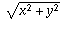, as illustrated by the right-angled triangle in the picture below.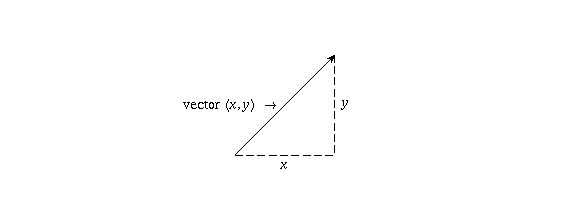The formula for the length of a 3-dimensional vector (x,y,z) is also easily obtained: you draw a right-angled triangle whose hypotenuse is the vector (x,y,z) and whose other two sides are (x,y,0) (whose length issince it's really just a 2-d vector) and (0,0,z) (whose length is just |z|). Applying Pythagoras's Theorem to this triangle givesThis formula for length readily generalizes to any number of dimensions. For example, a vector in 4-dimensional space can be given by four coordinates as (x,y,z,w), and its length is defined to be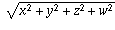by analogy to the length formula in two and three dimensions.

This, then, gives a definition of what the concept of length means in four and higher dimensions.

The other main geometric concept is that of angle. Again, we have an intuitive understanding of what it means in our 3-dimensional world, and it's not at first clear how to generalize it to higher dimensions.

However, if you apply the law of cosines to the three vectors u, v, and u- v illustrated below, the angle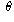between u and v satisfies the equationwhere | | denotes the length of a vector.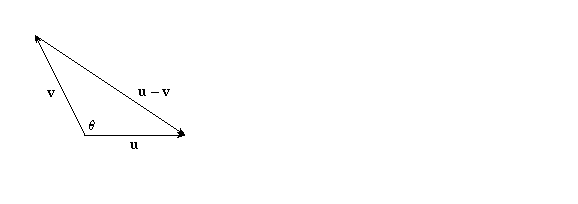Therefore, angle can be expressed in terms of length usingSince the length formulas make sense in any number of dimensions, so too does the notion of angle, if defined using this formula.

Finally, both length and angle are expressible in terms of a fundamental vector operation, the dot product: the dot product of two vectors (x,y,z,. . . ) and (a,b,c,. . . ) is defined to be the number xa + yb + zc + · · · obtained by multiplying together corresponding components and adding. This definition works for any number of dimensions. For example, the dot product of the two 5-d vectors (1,2,3,4,5) and (6,7,8,9,10) is

(1,2,3,4,5)·(6,7,8,9,10) = (1)(6) + (2)(7) + (3)(8) + (4)(9) + (5)(10) = 130.
Length relates to the dot product by the formula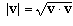, and angle by the formulaThe dot product is the fundamental operation from which all the geometric concepts can be defined. In fact, this is how mathematicians study abstract and complicated geometric spaces: any time, in any context, that you have an operation which satisfies the basic algebraic properties that the dot product satisfies, that operation defines a notion of geometry on the things you are studying, and you can employ geometric principles to study it, even though it may at first glance have seemed a very un-geometric situation!

This is exactly how geometry in four-, five-, and higher-dimensional Euclidean spaces is defined.

## Hypercubes

Shapes in higher dimensions can be expressed by giving equations among the coordinates, and a lot can be understood about them on that basis alone. For example, a 2-d square (with side length 1, located as shown below with respect to the coordinate axes) is the set of points (x,y) for which both x and y lie between 0 and 1.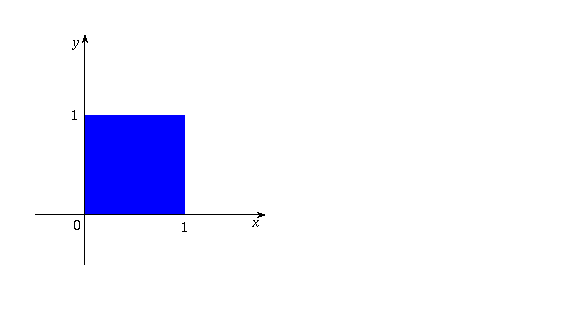A 3-d cube can be thought of as the set of points (x,y,z) for which x, y, and z each lie between 0 and 1.

In exactly the same way, a 4-d hypercube is the set of points (x,y,z,w) for which x, y, z, and w each lie between 0 and 1. The five-dimensional hypercube would be the set of points (x,y,z,w,u) for which x, y, z, w, and u each lie between 0 and 1. And so on: in n-dimensional space, the analogous thing to the hypercube is the set of points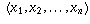such that eachsatisfies.

You can tell a lot about these objects just from that description. For example, let's ask ourselves, "what does the boundary look like?" We know that for an ordinary square, the boundary is just four line segments. For a cube, the boundary consists of six square faces. What about a hypercube?

Well, in order for a point (x,y,z,w) to be on the boundary, it means it's possible to move just a little bit away from that point and then be outside the hypercube: in other words, one of the coordinates would be less than 0 or greater than 1. The boundary case will be when one of the coordinates is exactly equal to 0 or 1.

There are eight possible such boundary conditions: for each of the four coordinates, there are two values (0 and 1) of that coordinate which give rise to a boudary piece.

This means that the boundary consists of eight pieces: the piece where x=0, the piece where x=1, the piece where y=0, and so on.

What do each of these boundary pieces look like? Well, consider for example the piece w=0. The remaining coordinates (x,y,z) are the only ones that are allowed to vary on the boundary, and they can range anywhere from 0 to 1. This is precisely the description of an ordinary three-dimensional cube.

Therefore, a hypercube's boundary consists of eight pieces, each of which is an ordinary cube.

If you carry out the same analysis in general, for n-dimensional space, you see that the n-dimensional hypercube has a boundary consisting of 2n pieces, each of which is an (n-1)-dimensional hypercube. (This is in complete agreement with what we already know about cubes and squares: the boundary of a cube is (2)(3)=6 squares, the boundary of a square is (2)(2)=4 line segments, and the boundary of a line segment is (2)(1)=2 endpoints).

You can also ask questions like, "how many vertices does a hypercube have?" A vertex occurs whenever all the coordinates are either 0 or 1. If there are n coordinates, with 2 choices for each, that givesdifferent vertices.

For example, when n=1 we have a line segment, which has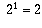vertices. When n=2 we have a square, which has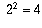vertices. When n=3 we have a cube, which hasvertices. When n=4 we have a hypercube, which has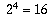vertices. And so on.

## Visualization

Of course, even though you can tell a lot about a hypercube just by looking at formulas involving coordinates, it's still nice to be able to try to visualize it in some way. People have developed quite a lot of skill at drawing 2-dimensional pictures of 3-dimensional objects. In the same way, one can try to draw a 3-dimensional picture of a 4-dimensional object like the hypercube. Mathematically, this is called a projection; a function which takes each point in the 4-dimensional object and maps it onto 3-dimensional space. The problem is, when putting something on paper (or on a computer screen) you have to make a further projection onto 2-dimensional space! That makes it very hard to draw pictures which accurately convey the 4-d reality. I will try to do the best I can, though.

One way to project from four dimensions to three dimensions is to just ignore one coordinate. That is, the four-dimensional point (x,y,z,w) could be drawn simply as the three-dimensional point (x,y,z). Under this projection, a hypercube just looks like a cube.

To understand why this is so, think about looking at an ordinary cube with one eye (no depth perception) from directly above one of the faces. It would look just like a flat square. So, when projecting from 3-d to 2-d by ignoring one coordinate, a cube looks like a square. Similarly, when projecting from 4-d to 3-d by ignoring one coordinate, a hypercube looks like a cube.

A slightly better way to view a cube is to look at it, still from above, but somewhat diagonally; then you get a 2-dimensional picture like the one shown below:What's happened is the top and bottom faces of the cube are projected onto slightly different locations in the plane: the 2-d picture of the top face (an ordinary square, shown in green) is shifted down and to the left from the 2-d picture of the bottom face (another ordinary square, shown in red). Now you can also see the edges joining the top and bottom faces (shown in blue).

Mathematically: this corresponds to taking the 3-d point (x,y,z) and drawing, in 2-d, not the point (x,y), but rather, the point (x,y) shifted down and to the left by an amount that depends on z. That is, the point (x,y,z) is drawn as the 2-d point (x-az, y-bz) (the scale factors a and b determine how much shifting to do). The above picture corresponds to a=b=1/2; thus the points (x,y,0) on the bottom face are drawn at (x,y), resulting in a (red) square with x, y ranging from 0 to 1, while the points (x,y,1) on the top face are drawn at (x-(1/2), y-(1/2)), resulting in a (green) square in which x and y range from -1/2 to 1/2.

You can do a similar thing with the hypercube: project it into 3-d, but shifting by an amount proportional to the fourth coordinate. That is, the 4-d point (x,y,z,w) is drawn at the 3-d point (x-aw, y-bw, z-cw) for some constants a, b, and c.

When you do that, you get a cube corresponding to the bottom "hyperface", drawn in red below (but remember what's drawn below is only a two-dimensional projection of the three-dimensional picture I'm talking about), and a shifted cube corresponding to the top hyperface, drawn in green below. Now you can visualize the remaining edges of the hypercube, drawn in blue, by joining up each vertex of the top-hyperface cube with the corresponding vertex of the bottom-hyperface cube.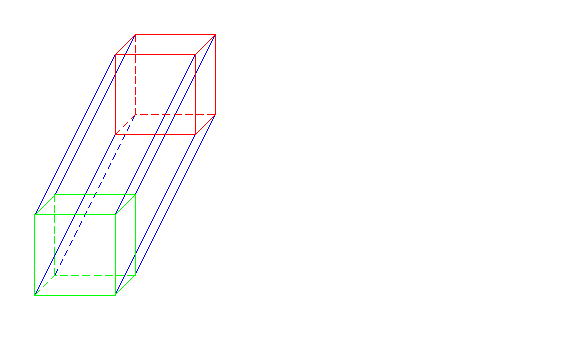Remember I stated above that the hypercube's boundary consists of 8 pieces, each of which is a cube. (These are what I'm calling the "hyperfaces"). Well, two of them (the red and green cubes) are visible in the picture above. But what of the other six? It's hard to see them from this picture, but if you try hard, I hope you can see that the four rightmost blue edges, together with the four rightmost red edges and the four rightmost green edges, form a third cube.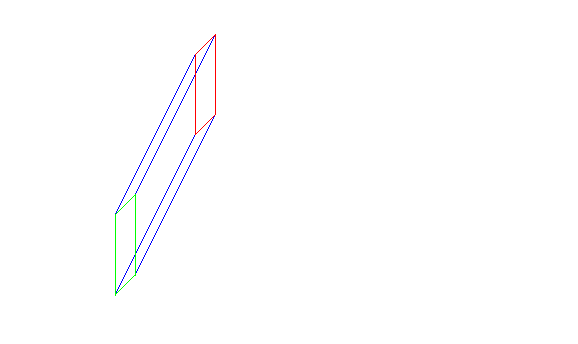The topmost blue, red, and green edges form the fourth boundary cube, the leftmost ones form the fifth, and the bottom ones form the sixth. The remaining two are harder to see, but the "frontmost" red square, the frontmost green square, and the blue edges joining them form the seventh boudary cube, thile the rearmost red square, the rearmost green square, and the blue edges joining them form the eighth one. To be able to see this well, you really have to do it from a 3-dimensional drawing; too much gets lost in the transition from 4-d to 3-d to 2-d to be able to see them clearly in the picture above.

Another way to project a cube onto a plane is to shrink the top of the cube a little bit, so when you look at it from above you see something like(to reconstruct the 3-d cube from this picture, think of pulling the inner square up from the paper, or out from the computer screen).

In other words, the top face of the cube is drawn in 2-d as a smaller square, inside the square with which the bottom face is drawn.

The analogous thing for hypercubes is to represent the top hyperface by one cube in 3-d and the bottom hyperface by a smaller cube inside the first cube. Then you can connect the corresponding vertices of the two cubes just as in the previous kind of projection. This is shown below.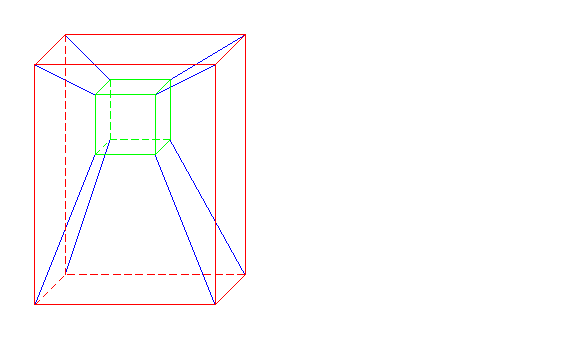The visualization of higher-dimensional hypercubes is much harder. You could do a similar kind of thing (e.g., project a 5-d hypercube into 4-d as one 4-d hypercube inside another with corresponding vertices joined, then draw each of those hypercubes as a cube inside another), but the pictures get very complicated very quickly and I'm not even going to attempt a drawing!

## Another 4-d solid: the 4-dimensional tetrahedron

Another symmetric 4-d "hypersolid" is the 4-dimensional analogue of a regular tetrahedron. Recall that a tetrahedron is a pyramid whose base and each of its sides are equilateral triangles:One way to visualize such a tetrahedron in 2-d is to think of cutting it open along the three edges leading up to the peak, then laying it flat. This gives the following picture:Observe that, in the picture, you have one triangle in the centre, then three other triangles, one attached to each of its sides. The pyramid is obtained by folding up those three triangles into the third dimension.

The 4-dimensional version can be visualized in the same way. You start with a solid tetrahedron in 3-d. To each of its four faces, you attach another tetrahedron. Now you've got a solid which it's a little hard to draw in 2-d, so I won't attempt it, but you might want to try making it with models:

1. First cut out of a piece of cardboard five copies of the picture above.

2. Fold along the three thick lines, until the tips of three outer triangles meet at the top, forming a pyramid. Attach them together with tape or glue.

3. Now you have five tetrahedrons. Choose one of them. Onto each of its four faces, glue one of the other four tetrahedrons.

Now you have one central tetrahedron with four others sticking out of it. The 4-dimensional tetrahedron is the hypersolid obtained by bending each of those sticking-out tetrahedra (in four dimensions, you have an extra dimension to bend them into) until they meet at a point. Obviously, you cannot do this in three dimensions, but you can in four.

The 4-dimensional tetrahedron can also be visualized in a manner similar to the second method described for visualizing the hypercube.

First lets understand how to visualize an ordinary 3-d tetrahedron in 2-d. If you stare directly at the top of it, with just one eye and no depth perception so that everything looks 2-dimensional, you see a picture like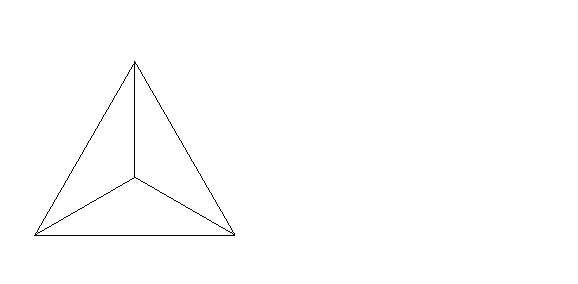where the peak of the tetrahedron is drawn inside the triangle representing the bottom face. This is a pretty good 2-d picture of a tetrahedron; you just have to imagine reaching in and pulling that central dot up and out of the page to get the real thing.

Observe that this picture consists of (a) a triangle, (b) a dot in the centre of the triangle, and (c) edges running between that dot and each of the triangle's three vertices.

In the same way, a 4-d tetrahedron can be visualized using the following 3-d model, illustrated below: (a) a tetrahedron (coloured red in the picture), (b) a dot in the centre of the tetrahedron, and (c) edges running between that dot and each of the tetrahedron's four vertices (coloured green).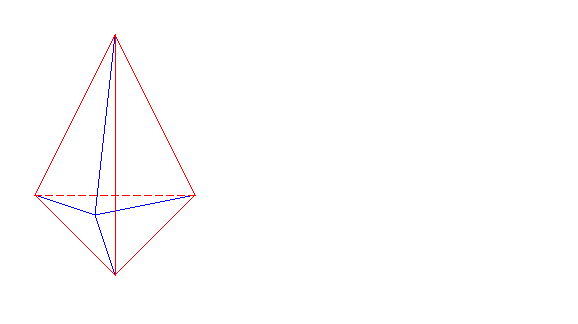There are analogues of the tetrahedron in every dimension, constructed by similar methods.

## Other hypersolids

You ask a very interesting question when you ask about other symmetrical solids in 4 and 5 dimensions. The well-known mathematician Donald Coxeter, part of the mathematics department at the University of Toronto, spent many years of his life precisely on this question, worked out the answer, and wrote extensively about it. I will not here go into the details of how one finds all the different higher-dimensional regular solids, but I hope that the above examples (of higher-dimensional analogues of the cube and the tetrahedron, which exist in every dimension) are of interest to you and to your son.

The reason the question is so interesting is that the theory of regular shapes changes as you change dimension. In 2 dimensions, you can have a regular shape (a polygon whose lengths and angles are all equal) with any number of sides. So, there are an infinite number of regular polygons: the equilateral triangle, the square, the regular pentagon, the regular hexagon, and so on.

However, in three dimensions, there are only five different kinds of regular solids, instead of an infinite number! They are the tetrahedron (a 4-sided solid whose faces are all equilateral triangles), the cube (a 6-sided solid whose faces are all squares), the octahedron (an 8-sided solid whose faces are all equilateral triangles), the dodecahedron (a 12-sided solid whose faces are all regular pentagons), and the icosahedron (a 20-sided solid whose faces are all equilateral triangles). There aren't any others.

The reason is quite interesting. Look at one of the vertices of a regular polyhedron (call it v). A certain number of faces (let's say, k faces) come together at v. The number k has to be at least 3 (because, as illustrated below, each face F has two edges, e and f, touching v, and F must be adjacent to some other face along e and also to some other face along f, making for a total of at least three faces touching v).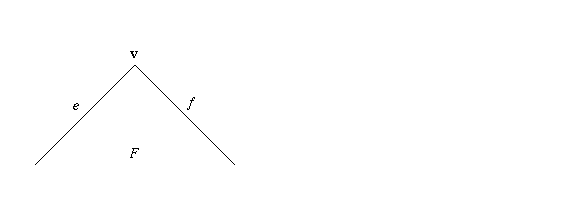However, there is also an upper limit on how large k can be, because the angles at any vertex must add up to less than 360 degrees (the reason is given below).

There are k of these angles. So, if the faces are equilateral triangles, in which the angles are 60 degrees, that means k times 60 must be less than 360, so k < 6. Therefore, the only possible regular polyhedra with equilateral triangles for faces are (a) one in which 3 faces meet at every vertex (this is the tetrahedron), (b) one in which 4 faces meet at every vertex (this is the octahedron), and (c) one in which 5 faces meet at every vertex (this is the icosahedron).

If the faces are squares, in which the angles are 90 degrees, we must have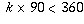, so k < 4. Thus k=3 is the only possibility, and the only possible regular polyhedron with squares for faces is one in which 3 faces meet at every vertex; this is the cube.

If the faces are pentagons, in which the angles are 108 degrees, we must have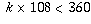, so k < 10/3, so k=3 is the only possibility. Therefore, the only possible regular polyhedron with pentagons for faces is one in which 3 faces meet at every vertex; this is the dodecahedron.

If the faces are polygons with 6 or more sides, the angles are 120 degrees or more, and we cannot have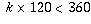unless k<3, which can't happen because there have to be at least three faces meeting at every vertex. Therefore, there is no regular polyhedron whose faces are hexagons, or any other polygon with 6 or more sides.

Now, what about 4 and higher dimensions? You can do a similar kind of analysis using solid angles instead of angles, and you find that there is only a limited, finite number of possibilities for the number of hyperfaces meeting at each vertex, depending on what the hyperfaces are (and, since the hyperfaces have to be regular solids, there are only five possibilities for those).

However, even if a particular combination is allowable according to the above analysis, there's no guarantee that there might not be some other reason ruling out the existence of such a regular hypersolid. So the work involved in figuring out exactly what kinds of regular polyhedra exist in 4 and higher dimensions is much more complicated than the analysis given above.

Nevertheless, I hope the above discussion gives you some idea of the kind of issues involved.

Finally, let me indicate why it is that the sum of the angles at a vertex of a regular polyhedron must add up to less than 360 degrees. If you take a two-dimensional picture of what happens at a vertex, as if you were looking through the vertex (directly toward the centre of the solid) without any depth perception, then in the two-dimensional picture the angles are larger than they are in the 3-d reality of the solid, and they add up to exactly 360 degrees. For instance, if you look at a corner of a cube, the real angles on the cube are 90 degrees, but the flattened out angles are 120 degrees: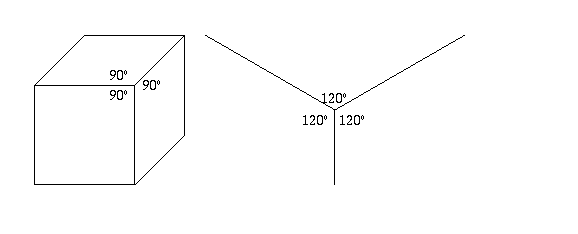This means the original angles in the solid must add up to less than 360 degrees.

The proof that the flattened-out angles are always less than the original ones uses vector algebra. If u and v are the flattened-out vectors corresponding to two of the edges, then the original 3-d vectors in the solid are these vectors plus a displacement vector w directed toward the centre of the solid.

Let a be the cosine of the flattened-out angle, and b the cosine of the original angle in the solid. If we scale things so that u and v have length 1, a is just u· v. However, b is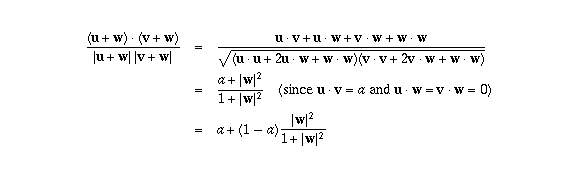which is greater than a (since a<1). So, the original angle in the cube has a larger cosine than the flattened-out angle, and hence it's a smaller angle.

[ Submit Your Own Question ] [ Create a Discussion Topic ]

This part of the site maintained by (No Current Maintainers)
Last updated: April 19, 1999
Original Web Site Creator / Mathematical Content Developer: Philip Spencer
Current Network Coordinator and Contact Person: Joel Chan - mathnet@math.toronto.edu

Navigation Panel: